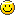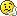# Traversing a geometry

VIVE L’AMOUR!
I am Pepe Le Pew. Zis is my first affair so, please, be kind. I’m shy but, uh, I’m willing…

I’d like to traverse a geometry and perform some actions based on the “name” and “path”.
I’ve tried three approaches, but none of them is really satisfactory to me.

1. An iterative function traversing nodes.

void MyNodeIter(TGeoVolume *vol) {
vol->GetGeoManager()->PushPath(); // save the original path

TGeoIterator next(vol);
TGeoNode *node;
TString path;

while ((node = next())) // daughters
{
next.GetPath(path);
vol = node->GetVolume();
vol->GetGeoManager()->cd(path.Data());
// do some “actions” for the current node
std::cout << node->GetName() << " "
<< vol->GetGeoManager()->GetPath() << " "
<< path << std::endl;
}

next.GetTopVolume()->GetGeoManager()->PopPath(); // restore the original path
}

void MyNodeIter(TGeoNode *node) {
MyNodeIter(node->GetVolume());
}

which is then called, for example, as

MyNodeIter(gGeomanager->GetTopVolume());

or as

MyNodeIter(gGeomanager->GetTopNode());

There are two problems here:
a. it does not work for the “starting node” (“actions” are performed for daughters only),
b. the “path” contains “A/B_0/C_0”, while “vol->GetGeoManager()->GetPath()” returns “/A_1/B_0/C_0” and if I do not “cd(path.Data())”, the “vol->GetGeoManager()->GetPath()” is never changing, it always returns “/A_1” (the “path” does change, however).

Can I somehow improve this function to perform my “actions” for the “starting node”, too?
Is the “PushPath” / “PopPath” logic doing what I’m “expecting”?

1. A recursive function traversing nodes.

void MyNodeRecur(TGeoNode *node) {

// I need to “CdDown(node)” but HOW?

// do some “actions” for the current node
std::cout << node->GetName() << " "
<< node->GetVolume()->GetGeoManager()->GetPath() << " "
<< std::endl;

// daughters now
for (Int_t i = 0; i < node->GetNdaughters(); i++)
MyNodeRecur(node->GetDaughter(i), name);

// I need to “CdUp()” but HOW?
}

which is then called, for example, as

MyNodeIter(gGeomanager->GetTopNode())

As one can see, my “actions” are performed for every node, including the “starting node”.

The problem with this function is that I do not know how to “CdDown” / “CdUp” and as a result the “GetPath()” always returns “/A_1” (and I do not know how to “GetPath(node)”).

1. A recursive function traversing volumes.

void MyVolRecur(TGeoVolume *vol) {

// I need to “CdDown(vol)” but HOW?

// do some “actions” for the current node
std::cout << vol->GetName() << " "
<< vol->GetGeoManager()->GetPath() << " "
<< std::endl;

// daughters now
for (Int_t i = 0; i < vol->GetNdaughters(); i++)
MyVolRecur(vol->GetNode(i)->GetVolume());

// I need to “CdUp()” but HOW?
}

void MyVolRecur(TGeoNode *node) {
MyVolRecur(node->GetVolume());
}

which is then called, for example, as

MyVolRecur(gGeomanager->GetTopVolume());

or as

MyVolRecur(gGeomanager->GetTopNode());

As one can see, my “actions” are performed for every node, including the “starting node”.

The problem with this function is that I do not know how to “CdDown” / “CdUp” and as a result the “GetPath()” always returns “/A_1” (and I do not know how to “GetPath(vol)”).

I am stupid. No?
Pepe Le Pew.

Salut Pepe,

Well, that’s long, but I am not sure I understood what you are trying to do exactly, so I will try to give you some hints for the methods you use:

• TGeoManager::cd(path) is used to make the navigator point to a physical node in the geometry. It make sense to use this method if you want to do coordinate or direction vectors conversions between the master reference frame and the one local to a given node. Can be used also to retrieve the global matrix associated to a path in geometry.

• Push/PopPath are used to backup/restore a given geometry state during track propagation. These methods are a bit expensive and allocating memory (Push) so it make sense use them only for this purpose (never do a push for all paths in the geometry)

• TGeoIterator is supposed to iterate all the node hierarchy of a volume. This is why the path returned by GetPath() starts with a volume name instead of a node name. This is usable for any volume, not only the top one. If you really need to make a match between the returned path and a full one (starting with TOP_1) you can add _1 to the top volume name, but only when the iterator is applied to it

• A node or volume are logical objects that know nothing about their parent (container). So you can never CdUp() based on this info. The same node/volume may have several parents.

• Most of the thinks you seem to try (investigating the geometry hierarchy) can be done directly from the iterator. You should maybe point out what are actually the “actions” you need to perform.

Cheers,

VIVE L’AMOUR!
Please find attached a set of recursive and iterative routines which traverse a geometry in order to find its specific parts (either “volumes” or “nodes”) identified by their “names”.I didn’t really test them very extensively but they seem to work for me.I wonder how “general purpose” they are - can you think of “cases” when they will fail?A pitiful case, am I not?
Pepe Le Pew.
TestGeometryScan.cxx (13.3 KB)